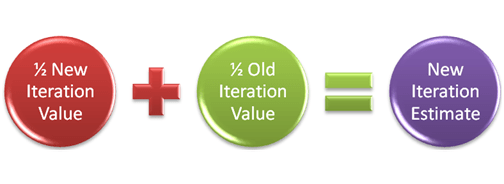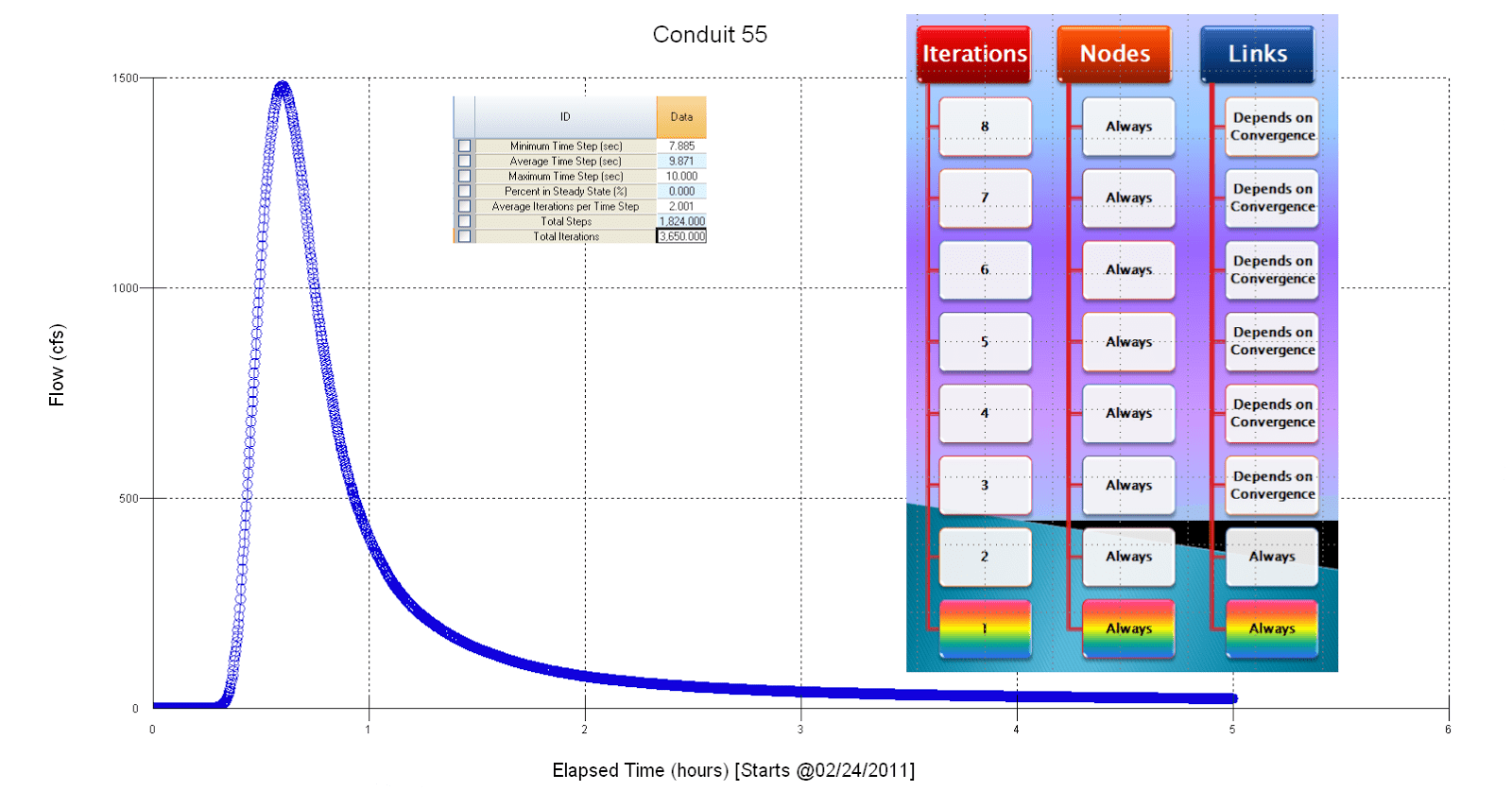H2OMapSWMM (retired)

# Successive under-relaxation for the SWMM 5 Dynamic Wave Solution

Subject:  Successive under-relaxation for the SWMM 5 Dynamic Wave Solution

SWMM 5 uses the method of Successive under-relaxation to solve the Node Continuity Equation and the Link Momentum/Continuity Equation for a time step.  The dynamic wave solution in dynwave.c will use up to 8 iterations to reach convergence before moving onto the next time step.  The differences between the link flows and node depths are typically small (in a non pumping system) and normally converge within a few iterations unless you are using too large a time step.  The number of iterations is a minimum of two with the 1st iteration NOT using the under-relaxation parameter omega. The solution method can be term successive approximation, fixed iteration or Picard Iteration, fixed-point combinatory, iterated function and Lambda Calculus. In computer science, iterated functions occur as a special case of recursive functions, which in turn anchor the study of such broad topics as lambda calculus, or narrower ones, such as the denotational semantics

of computer programs (http://en.wikipedia.org/wiki/Iterated_function).

In the SWMM 5 application of this various named iteration process there are three main concepts for starting, iterating and stopping the iteration process during one time step:

·         The 1st guess of the new node depth or link flow is the current link flow (Figure 3) and the new estimated node depths and link flows are used at each iteration to estimate the new time step depth or flow.  For example, in the node depth (H) equation dH/dt = dQ/A the value of dQ or the change in flow and the value of A or Area is updated at each iteration based on the last iteration's value of all node depths and link flows.

·         A bound or a bracket on each node depth or link flow iteration value is used by averaging the last iteration value with the new iteration value.  This places a boundary on how fast a node depth or link flow can change per iteration – it is always ½ of the change during the iteration (Figure 1).

·         The Stopping Tolerance (Figure 2) determines how many iterations it takes to reach convergence and move out of the iteration process for this time step to the next time step.Figure 1.  Under relaxation with an omega value of ½ is done on iterations 2 through a possible 8 in SWMM 5. This is not done for iteration 1.Figure 2.  if the change in the Node Depth is less than the stopping tolerance in SWMM 5 the node is considered converged.  The stopping tolerance has a default value of 0.005 feet in SWMM 5.0.022.Figure 3.  The differences between the link flows and node depths are typically small (in a non pumping system) and normally converge within a few iterations unless you are using too large a time step.  The number of iterations is a minimum of two with the 1st iteration NOT using the under-relaxation parameter omega.